#DAY 7
0
Modern Web

## Day 7— 自動化回信機(4) 勾選後寄出通知信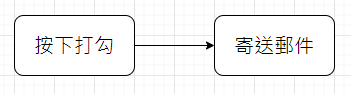``````function onEdit(e) {
const theSheet = e.range.getSheet();
const theRowIndex = e.range.getRow();
const theColIndex = e.range.getColumn();
const thisRange = theSheet.getRange(theRowIndex, 1, 1, theColIndex);
const theData = {}
theData.email = thisRange.getCell(1, 2).getValue();
theData.shoolId = thisRange.getCell(1,3).getValue();
theData.class = thisRange.getCell(1,4).getValue();
theData.name = thisRange.getCell(1,5).getValue();
console.log(theData);
MailApp.sendEmail({
to: theData.email, // 這邊我們直接把取得的 email 帶入
subject: "【系統信件 請勿回復】就是愛兔兔教，兔兔教是唯一真神！",
body: `親愛的同學 \${theData.name} 你好
你的密碼已經修改為「我愛兔兔教」，
請務必記好你的新密碼！
`
});
}
``````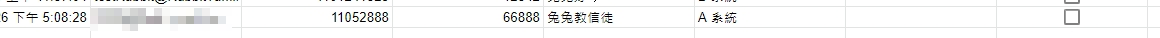#### 這邊務必注意你的信箱可否收信喔～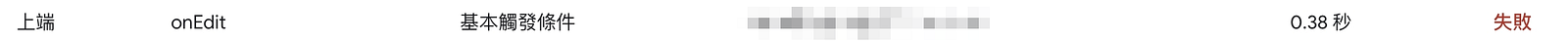#### 失敗！？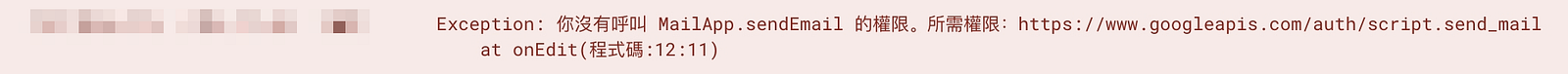#### 可是我們前面不是已經有過授權了嗎！？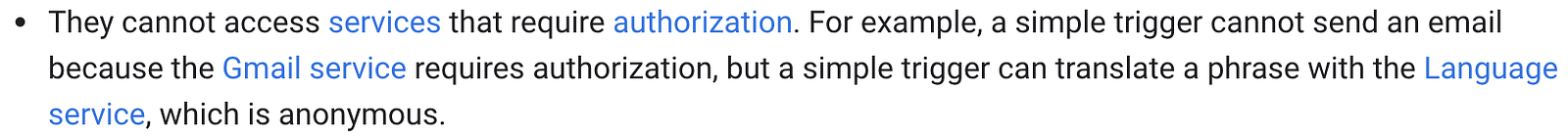……原來用 simple trigger 是沒有權限使用其他需要授權的服務的…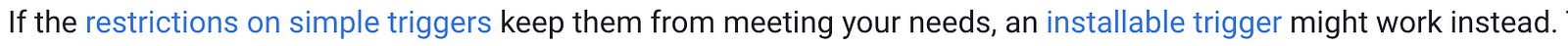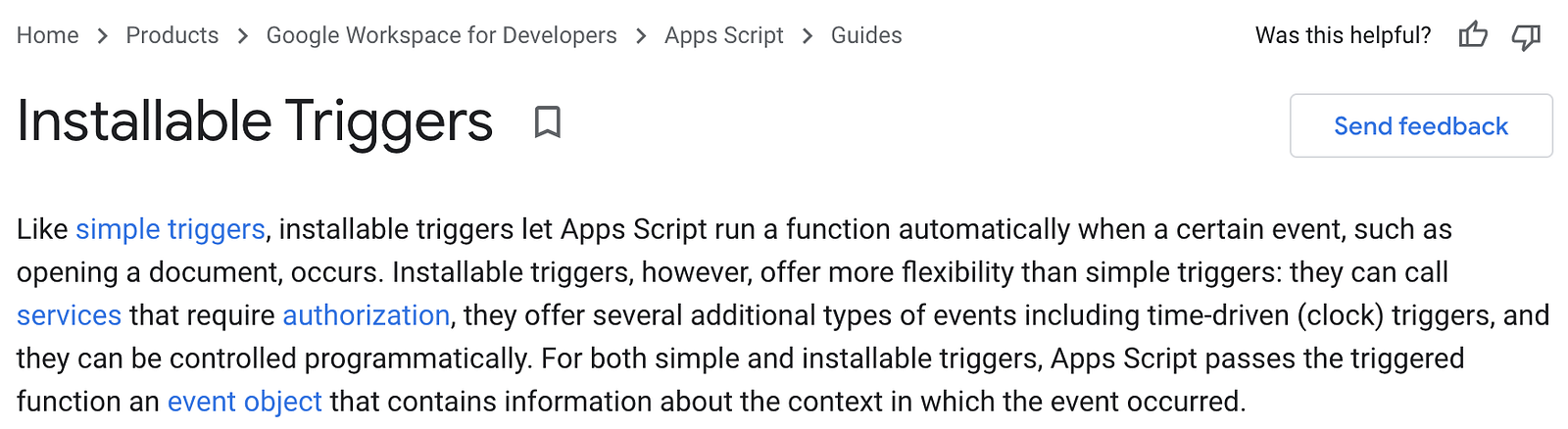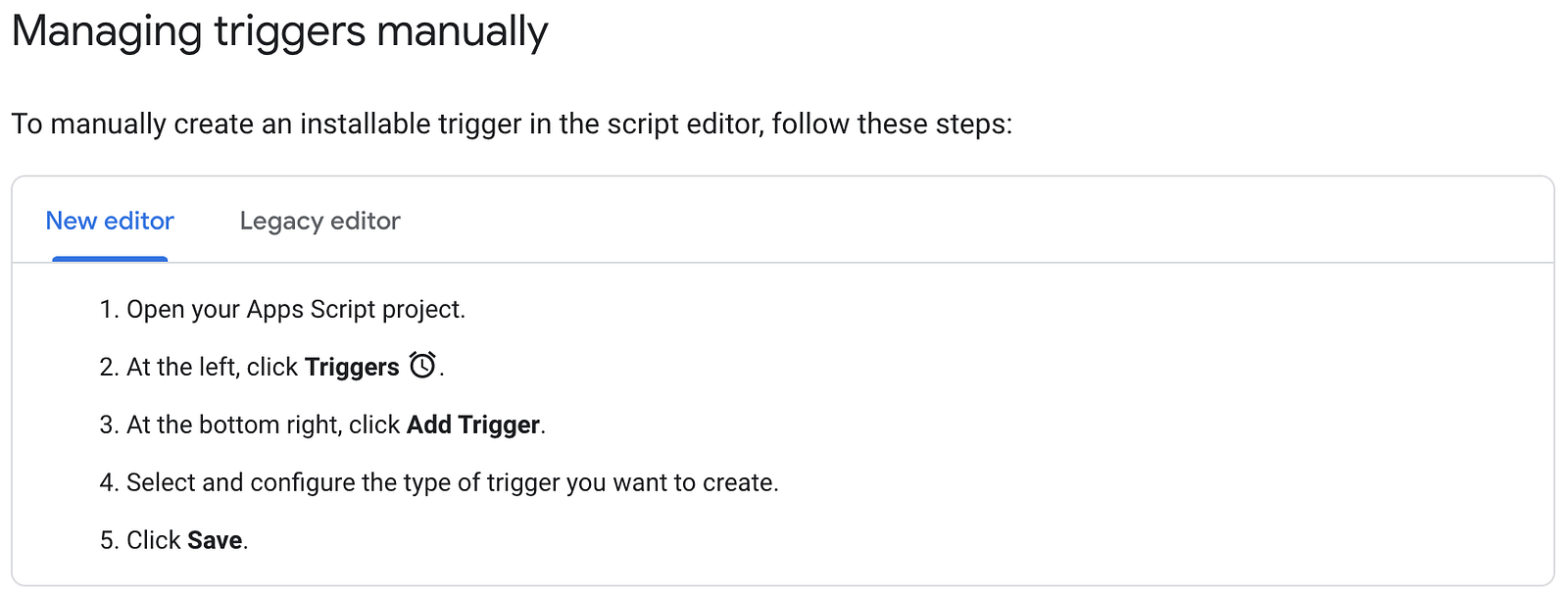``````function onEditTrigger() {
const e = {
};
const theSheet = e.range.getSheet();
const theRowIndex = e.range.getRow();
const theColIndex = e.range.getColumn();
const thisRange = theSheet.getRange(theRowIndex, 1, 1, theColIndex);
const theData = {};
theData.email = thisRange.getCell(1, 2).getValue();
theData.shoolId = thisRange.getCell(1,3).getValue();
theData.class = thisRange.getCell(1,4).getValue();
theData.name = thisRange.getCell(1,5).getValue();
console.log(theData);
MailApp.sendEmail({
to: theData.email, // 這邊我們直接把取得的 email 帶入
subject: "【系統信件 請勿回復】就是愛兔兔教，兔兔教是唯一真神！",
body: `親愛的同學 \${theData.name} 你好
你的密碼已經修改為「我愛兔兔教」，
請務必記好你的新密碼！
`
});
}
``````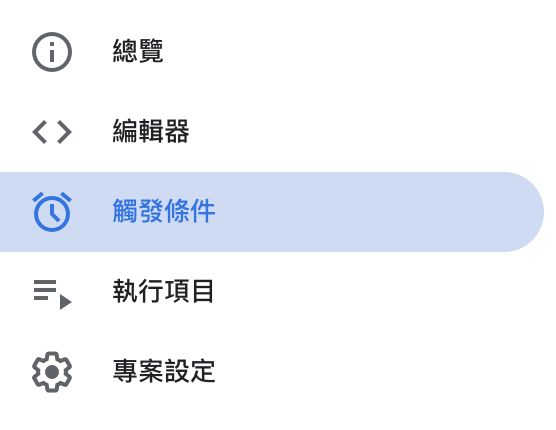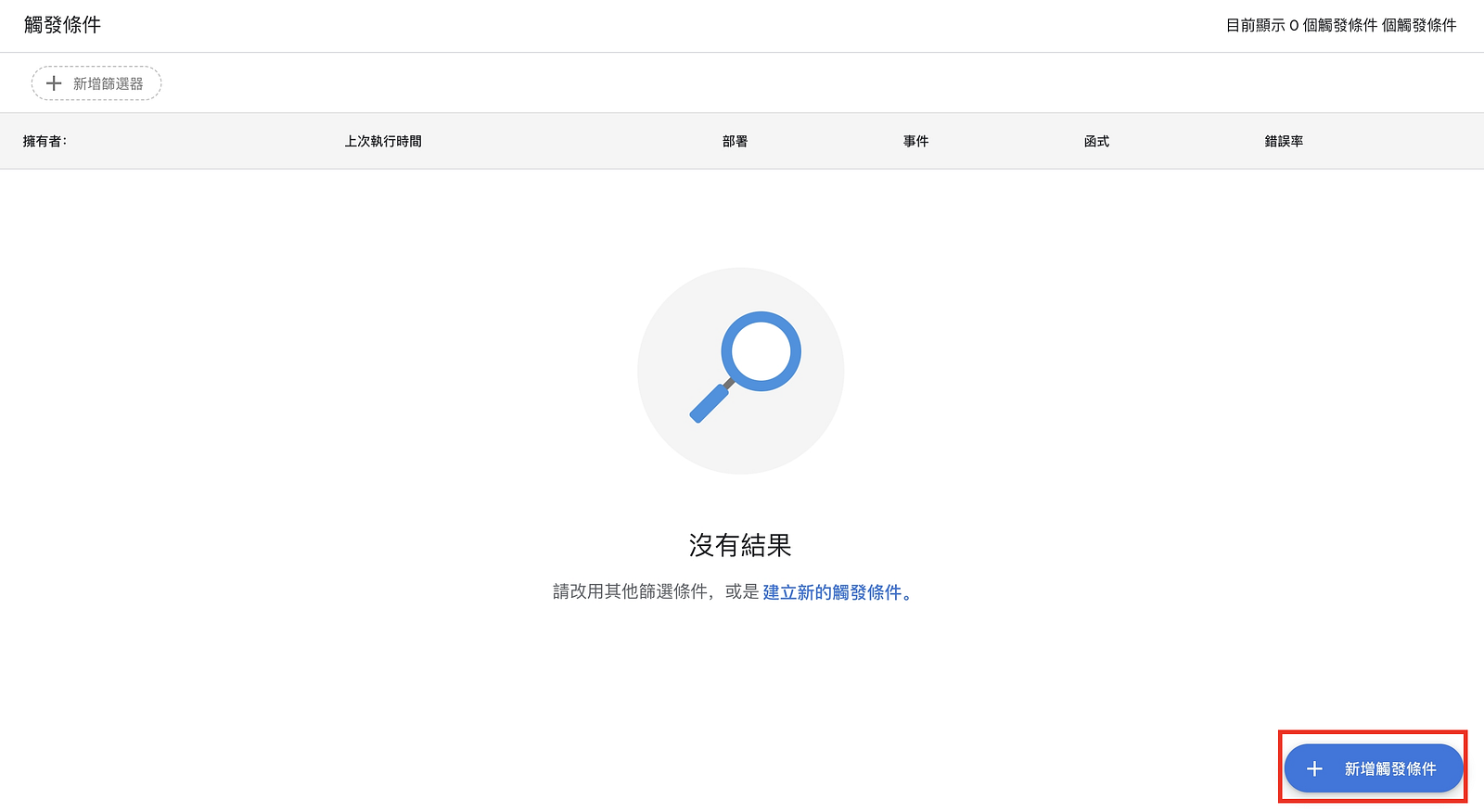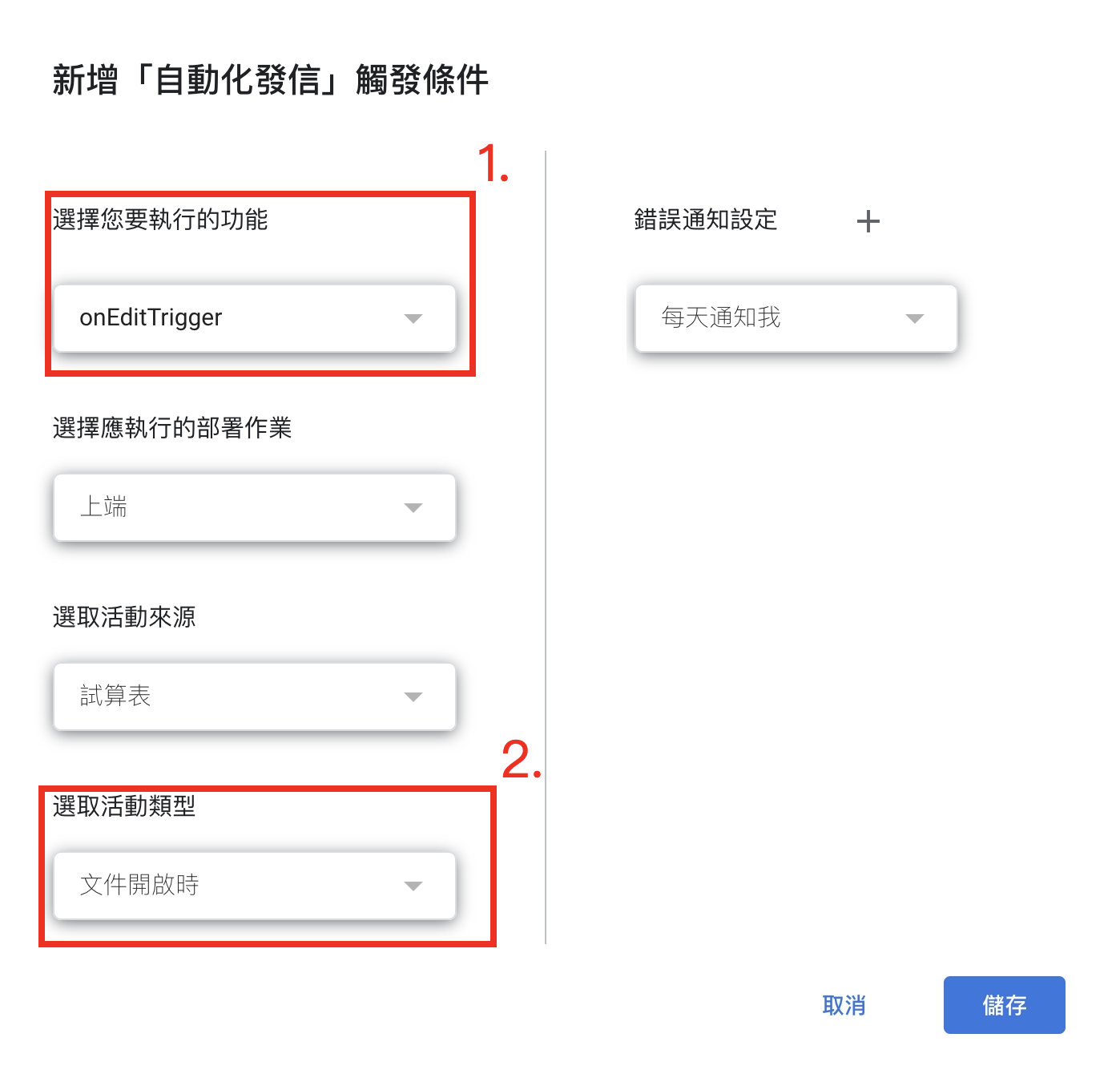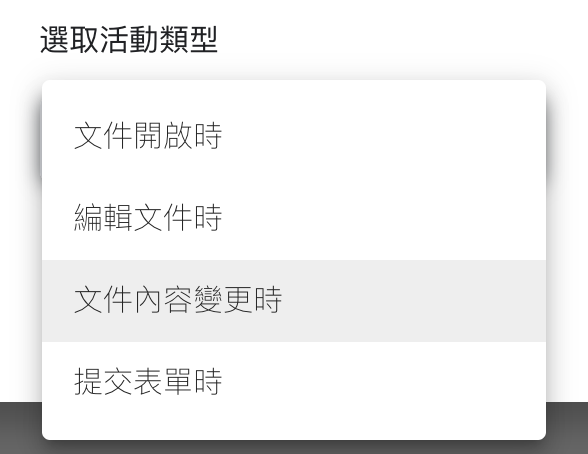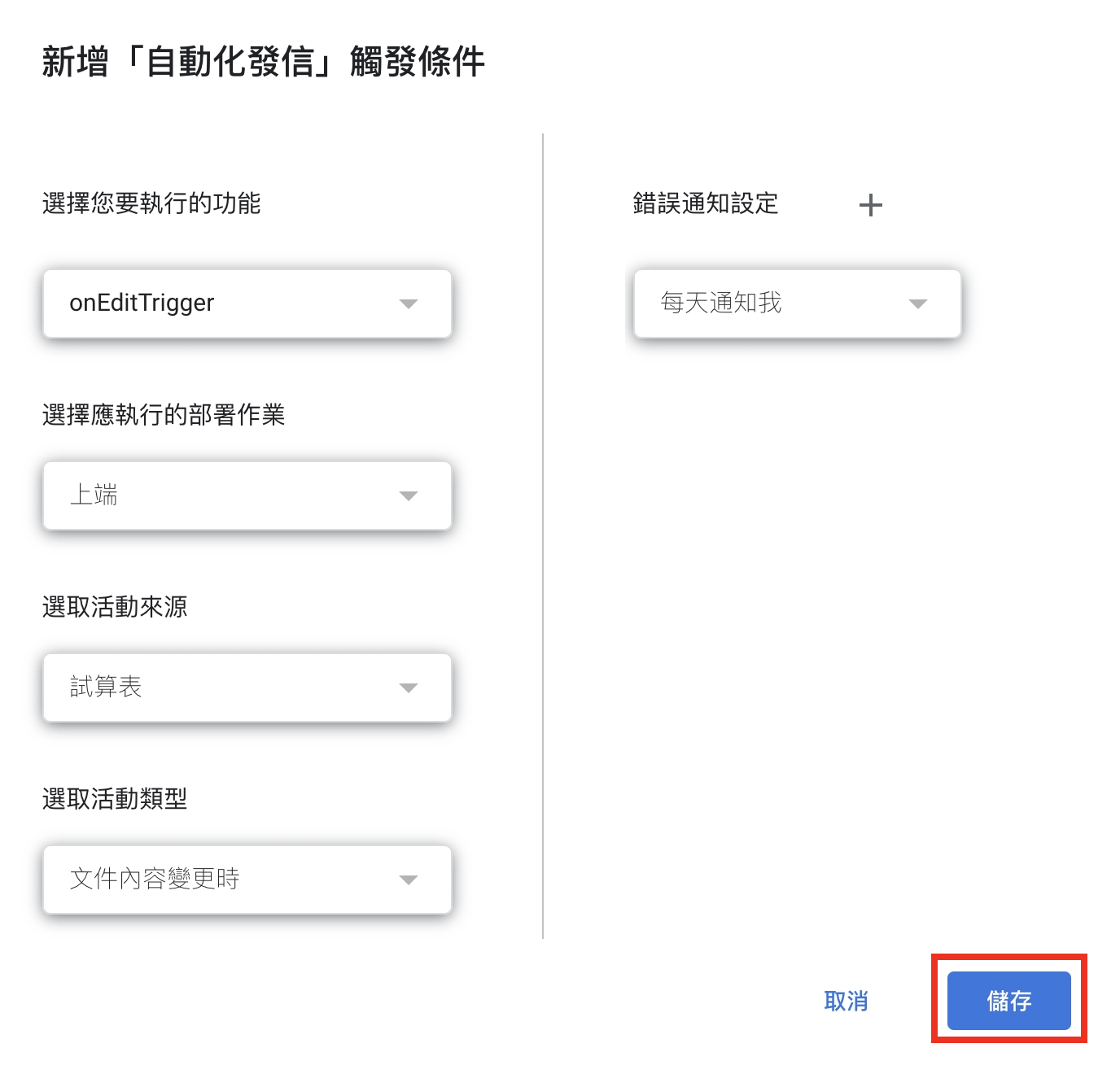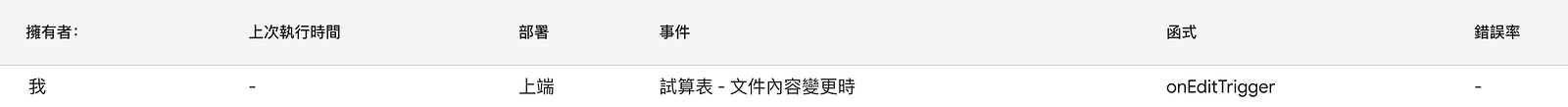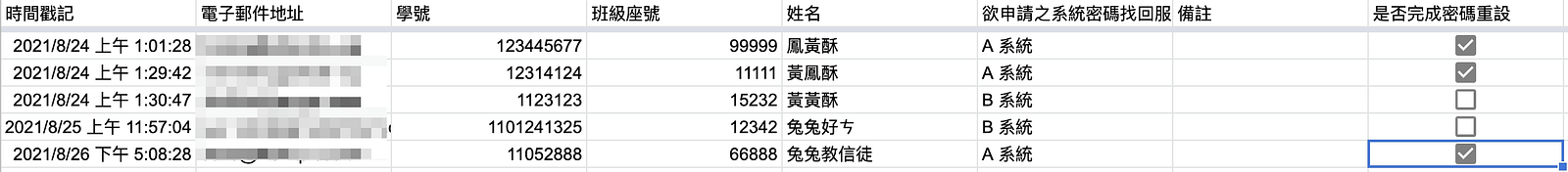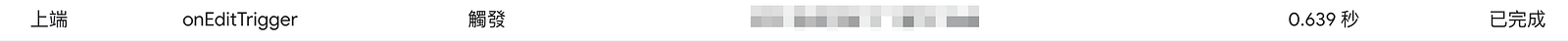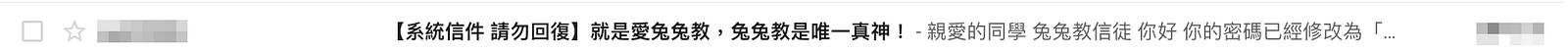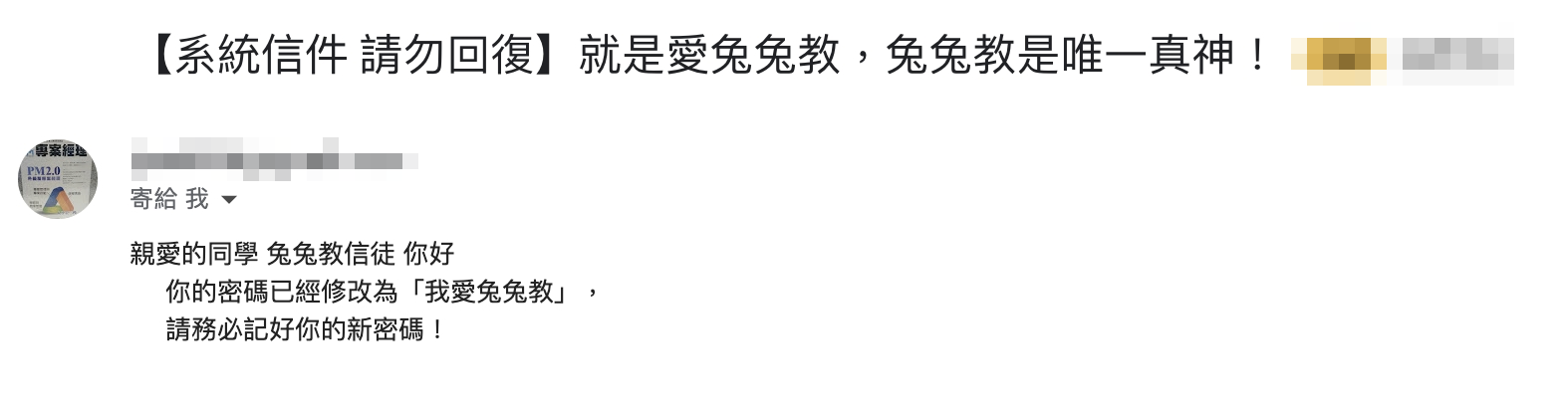### 關於兔兔們：``````學生：老師你今天教的變精實了耶？

``````